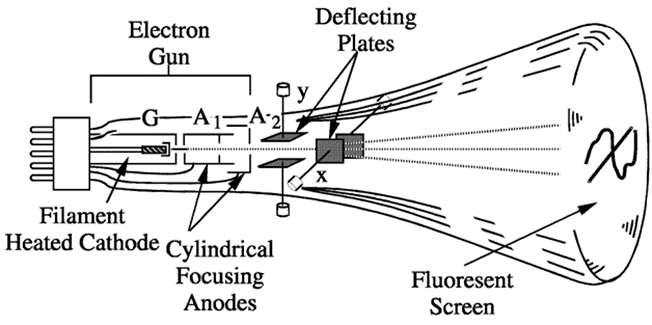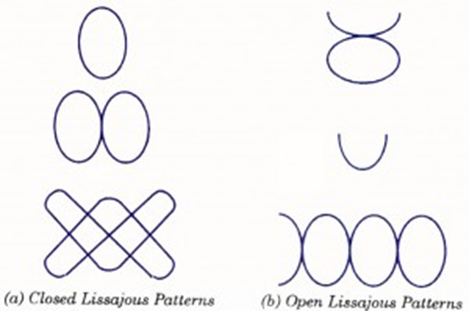Question:
Published on: 28 June, 2022

What is lissajous pattern? Explain how phase & frequency can be measured using this figures.

Cathode Ray Oscilloscope (CRO) is very important electronic device. CRO is very useful to analyze the voltage wave form of different signals. The main part of CRO is CRT(Cathode Ray Tube). A simple CRT is shown in figure below-When both pairs of the deflection plates (horizontal deflection plates and vertical deflection plates) of CRO (Cathode Ray Oscilloscope) are connected to two sinusoidal voltages, the patterns appear at CRO screen are called the Lissajous pattern. Shape of these Lissajous pattern changes with changes of phase difference between signal and ration of frequencies applied to the deflection plates (traces) of CRO. Which makes these Lissajous patterns very useful to analysis the signals applied to deflection plated of CRO.

These lissajous patterns have two Applications to analysis the signals To calculate the phase difference between two sinusoidal Signals having same frequency. To determine the ratio frequencies of sinusoidal signals applied to the vertical and horizontal deflecting plates.

MEASUREMENT OF PHASE DIFFERENCE

We have discussed that when two sinusoidal voltage signals of equal frequency having some phase difference are applied to the deflection plates of CRO, a straight line or an ellipse appears on the screen. In the case of straight line appearing on the screen, phase angle difference would be zero or 180° but in case of an ellipse we will have to use a formula for determination of phase difference.

Let there be two sinusoidal voltage signals given by

$$v_h = V_h Sin ωt$$

$$v_v = V_v Sin (ωt+Ф)$$, where Ф is the phase difference.

Since deflection is directly proportional to the amplitude of voltage

So, $$d_h = D_h Sin ωt$$

$$d_v = D_v Sin (ωt+Ф)$$

At time t=0, values of $$d_h$$ and $$d_v$$ are $$d_{h0}$$ = 0 and $$d_{v0} = D_v Sin Ф$$

So, $$Sin Ф = d_{v0}/D_v$$

Graphical meaning of $$d_{v0}$$ and $$D_v$$ are shown in the figure. Thus the phase difference between two sinusoidal voltages of equal frequency can be determined by measuring $$d_{v0}$$ and $$D_v$$.

In the given figure $$V_v$$ is shown by leading $$V_h$$ by a phase angle Ф. If the situation is reversed and $$V_h$$ leads $$V_v$$ by phase angle Ф, then again same ellipse would appear on the screen. Because of this fact we can determine only the phase angle between two sinusoidal voltages. It does not indicate which one is leading and which one is lagging.

MEASUREMENT OF FREQUENCY OF A VOLTAGE SIGNAL

The patterns obtained on CRO screen and discussed in previous sections are called the Lissajous patterns. A Lissajous pattern is a pattern which is stationary on the screen of a CRO. It means that the spot traces out the same pat¬tern for every cycle of a voltage signal. As we have already stud¬ied that the ratio of frequencies of vertical and horizontal voltage signals should be a rational or fractional number to have steady pattern. So the condition for having a Lissajous pattern on the CRO screen is

$$F_y/F_x=A/B$$

where A and B are integers.

Lissajous patterns are usually of two types. First one is closed Lissajous pattern and has no free end. Second one is open Lissajous pattern and has free ends. Both types of Lissajous patterns are shown in figure.

In a Lissajous pattern ratio of frequency of vertical signal to the frequency of horizontal signal is equal to the ratio of positive Y-peaks to positive X-peaks in that pattern.

$$F_y / F_x$$ = Positive Y – peaks in pattern / Positive X – peaks in pattern

Thus by counting the positive Y-peaks and X-peaks on a Lissajous pattern, ratio of frequencies of two voltage signals can be determined. In case of an open lissajous pattern, free end is treated as half peak. This will be clear from examples.

Voltage signal of unknown frequency is applied to the vertical deflection plates and horizontal deflection plates are supplied by an accurately calibrated variable frequency source. Frequency of the variable frequency source is adjusted until a stationary pattern appears on the screen. Now by reading the value of frequency of horizontal signal, with the help of calibrated scale, frequency of voltage signal applied to vertical deflection plates can be known.Lissajous Patterns

In case single loop stationary pattern is obtained the frequency of the sinusoidal voltage applied to vertical deflection pates is the same as that of the voltage applied to horizontal deflection plates. In case complex Lissajous figure is obtained, the frequency of alternating voltage may be determined using the relation

Points of tangency to a vertical line / Points of tangency to a horizontal line = $$ω_x/ω_y = f_x/f_y$$

One point, very interesting, to know is that sometimes we may have different types of patterns on the CRO screen for the voltage signals of the given frequencies, as shown in figure.

In both of the figures, the ratio of Y-peaks to X-peaks is equal so in both cases, ratios of fre-quencies of vertical and horizontal signals are same. But appearance of Lissajous patterns is different owing to different phase difference of voltage signals applied to vertical arid horizontal deflection plates.

CRO is not a precision instrument for measuring frequency of an alternating voltage because the accuracy depends directly on the accuracy of calibrated scale of variable frequency source, which is usually a few percent. It is used for rough estimate of frequency or when voltage waveform is so complex that a frequency counter would not operate reliably.

Random questions